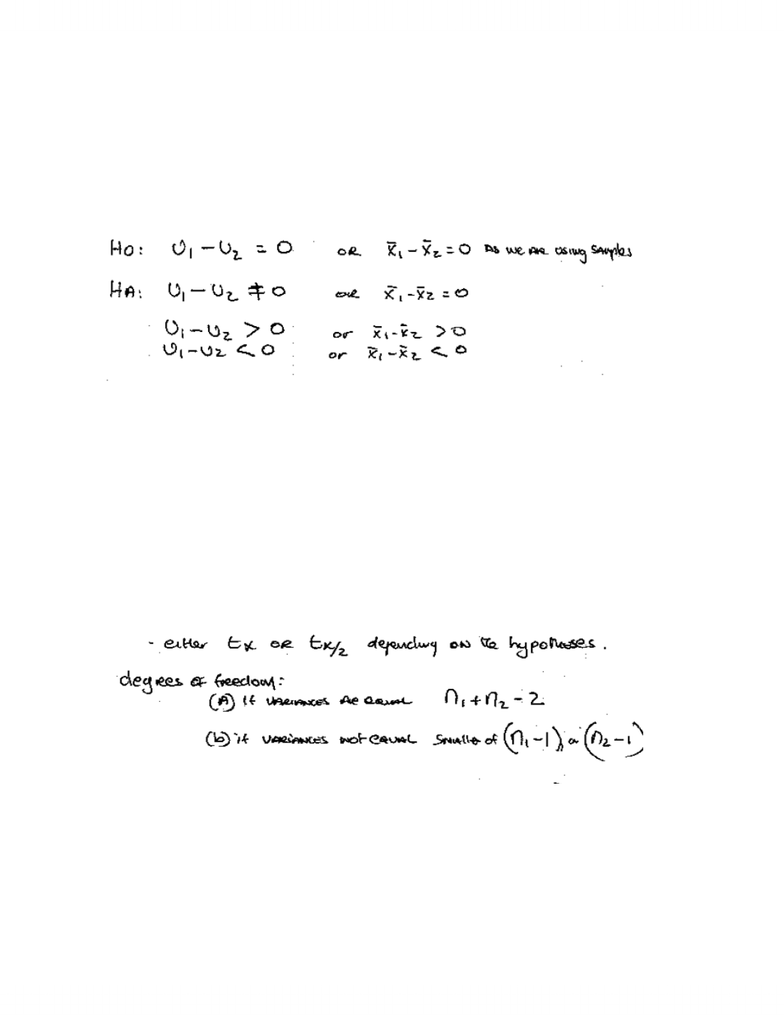Study Guides (390,000)
CA (150,000)
UTSG (10,000)
Geography (100)
Midterm

# Two Sample Means Test

Department
Geography
Course Code
GGR270H1
Professor
Damian Dupuy
Study Guide
Midterm

This preview shows page 1. to view the full 4 pages of the document.1
Two Sample Means Test
¾ Used when the objective is to compare two samples
¾ You are testing whether there is a significant difference between two samples
¾ If the sample differences are significant, then statistical inference allows the researcher
to conclude that the samples are drawn from different populations.
1. Hypotheses
*HQHUDOIRUP«
H0: No significant difference between the mean of sample 1 and the mean of sample 2.
Therefore, the samples are drawn from the same population.
HA: There is a significant difference between the mean of sample 1 and the mean of
sample 2. Therefore, the samples are drawn from different populations.
2. Critical Value
Use the t distribution,
3. Test Statistic
Use the t test.
Two forms of this test arise:
(a) Variances are equal
(b) Variances are unequal
*HQHUDOIRUP«
www.notesolution.com